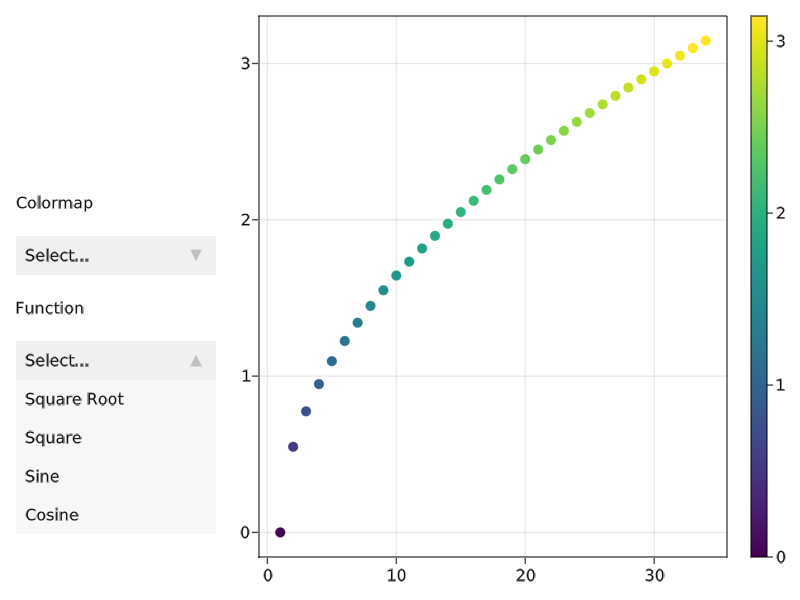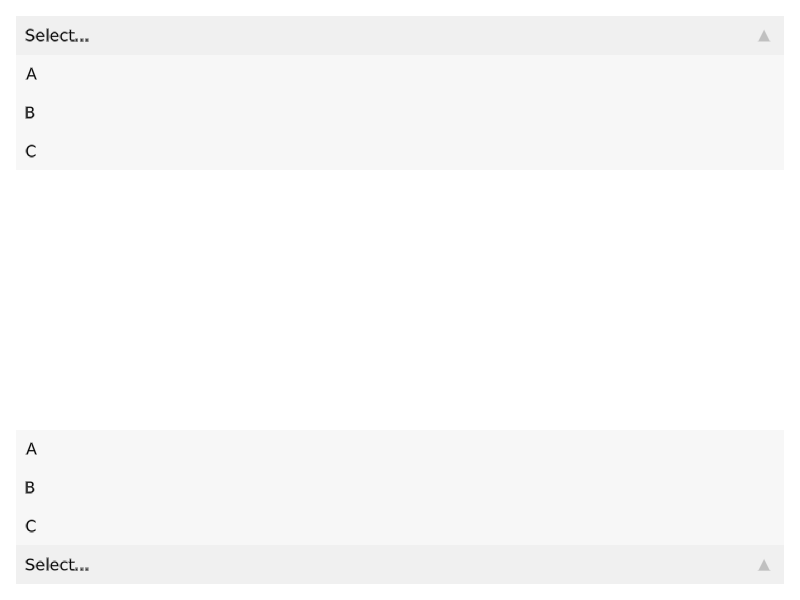A dropdown menu with options, where each element's label is determined with optionlabel(element) and the value with optionvalue(element). The default behavior is to treat a 2-element tuple as (label, value) and any other object as value, where label = string(value).

The attribute selection is set to optionvalue(element) when the element's entry is selected.

using GLMakie
fig = Figure()

menu = Menu(fig, options = ["viridis", "heat", "blues"])

funcs = [sqrt, x->x^2, sin, cos]

menu2 = Menu(fig, options = zip(["Square Root", "Square", "Sine", "Cosine"], funcs))

fig[1, 1] = vgrid!(
Label(fig, "Colormap", width = nothing),
menu,
Label(fig, "Function", width = nothing),
menu2;
tellheight = false, width = 200)

ax = Axis(fig[1, 2])

func = Node{Any}(funcs)

ys = @lift(\$func.(0:0.3:10))
scat = scatter!(ax, ys, markersize = 10px, color = ys)

cb = Colorbar(fig[1, 3], scat)

on(menu.selection) do s
scat.colormap = s
end

on(menu2.selection) do s
func[] = s
autolimits!(ax)
end

menu2.is_open = true

figYou can change the direction of the menu with direction = :up or direction = :down. By default, the direction is determined automatically to avoid cutoff at the figure boundaries.

using GLMakie

fig = Figure()

menu = Menu(fig[1, 1], options = ["A", "B", "C"])
menu2 = Menu(fig[3, 1], options = ["A", "B", "C"])

menu.is_open = true
menu2.is_open = true

fig